Ex 1.4

Chapter 1 Class 10 Real Numbers
Serial order wise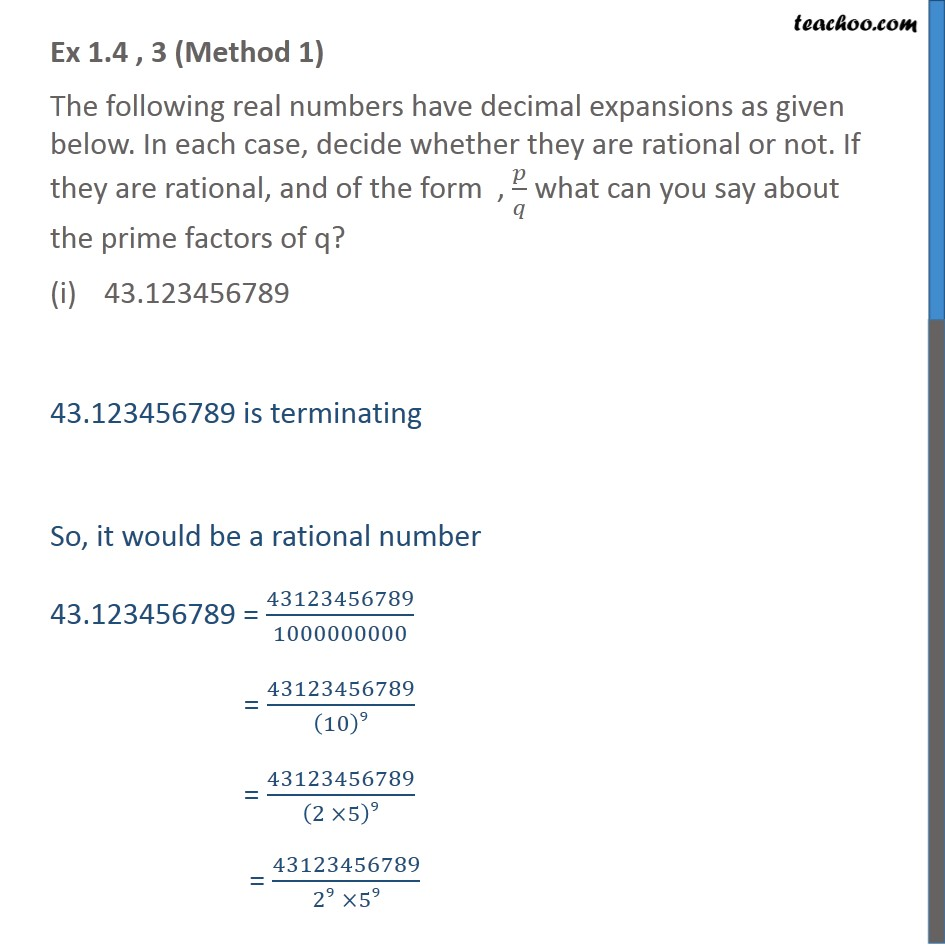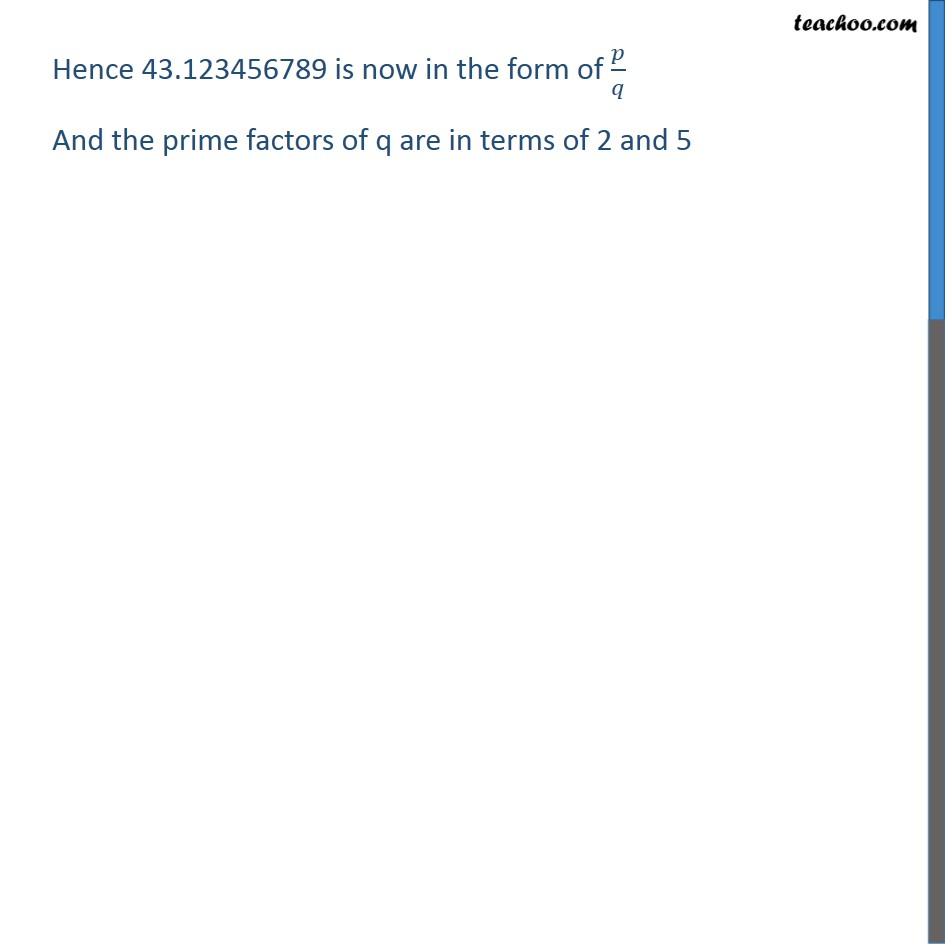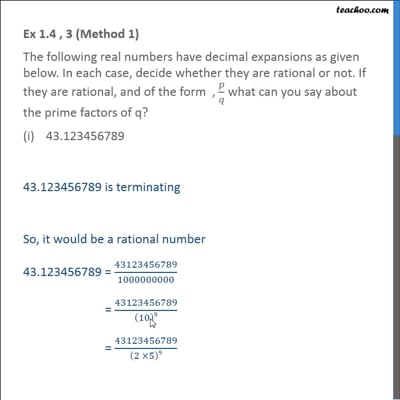This video is only available for Teachoo black users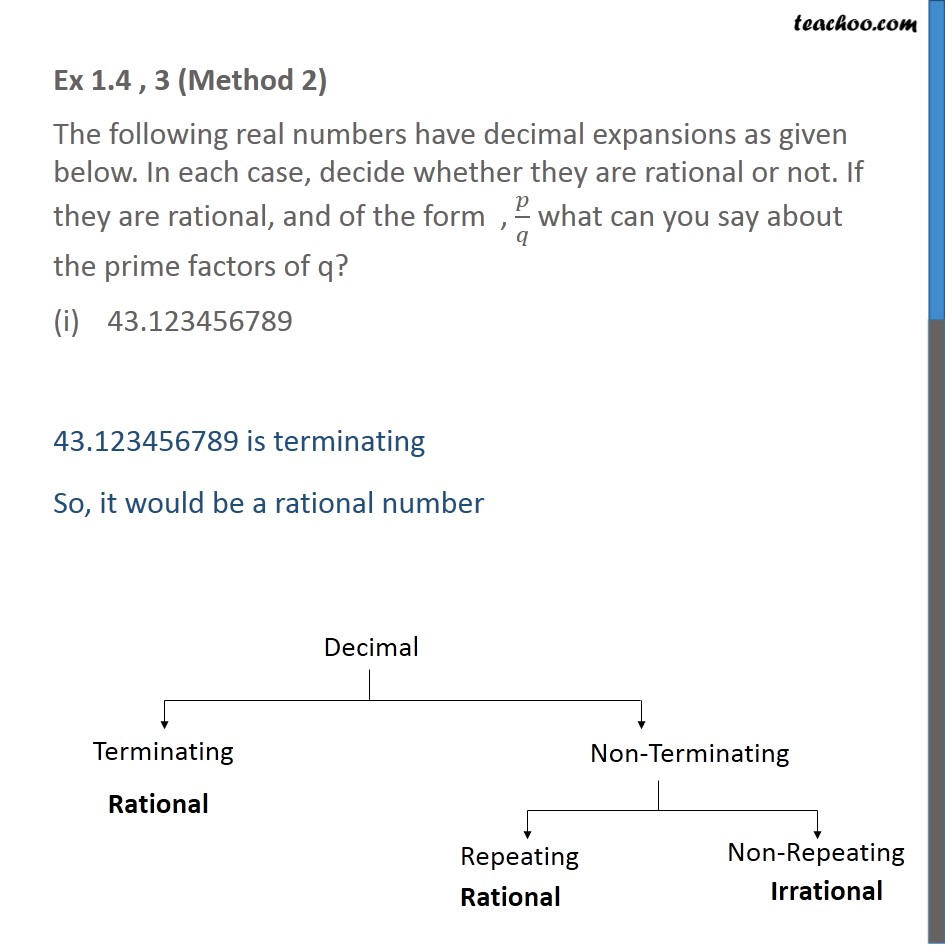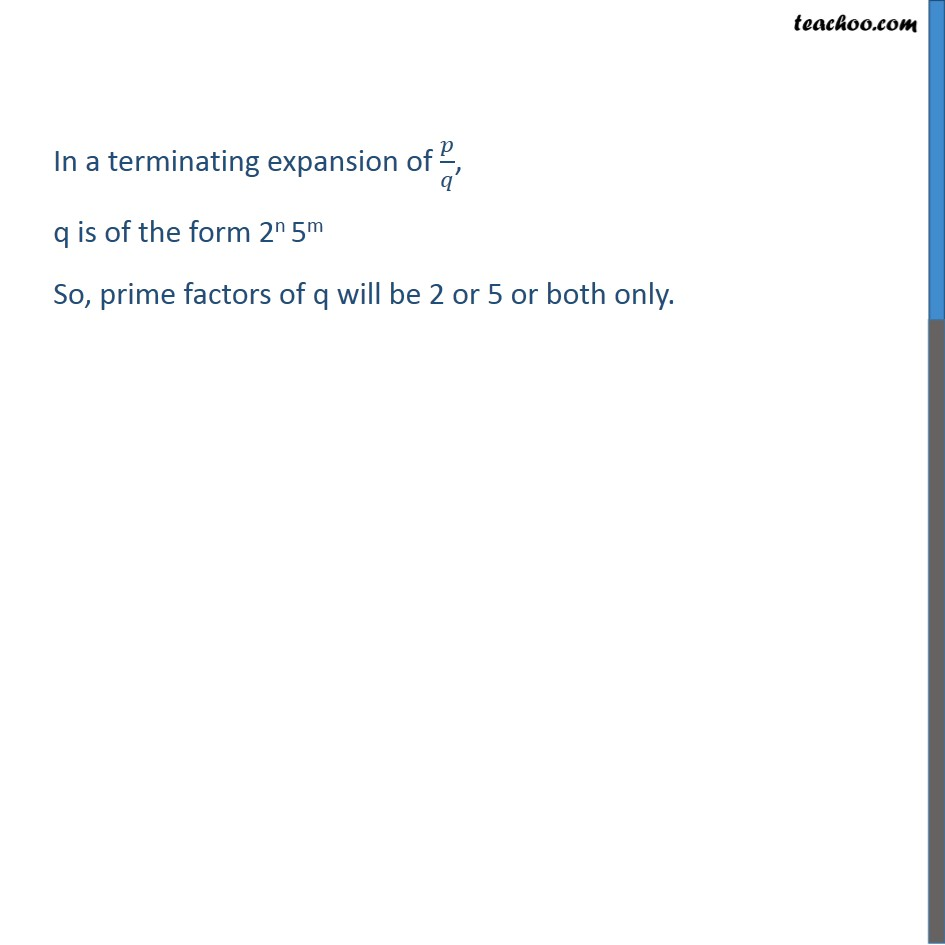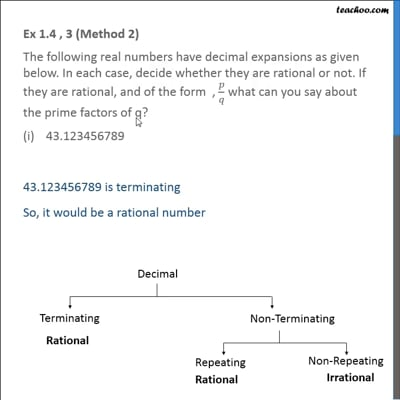This video is only available for Teachoo black users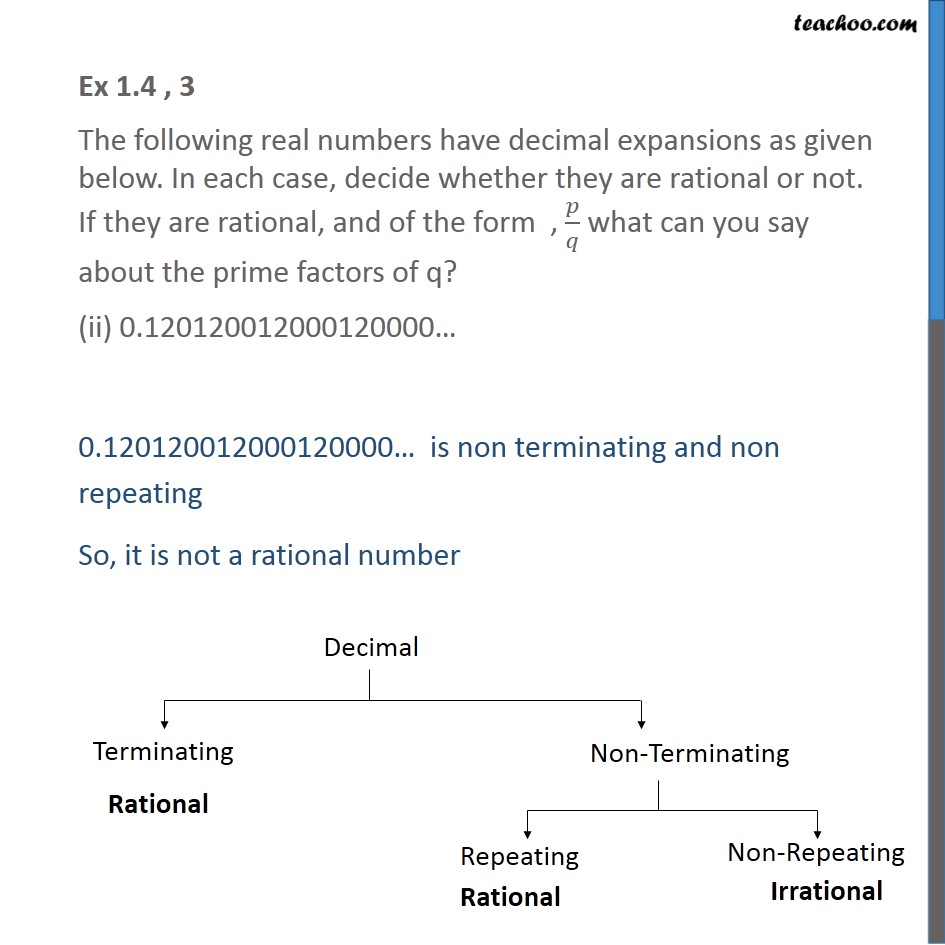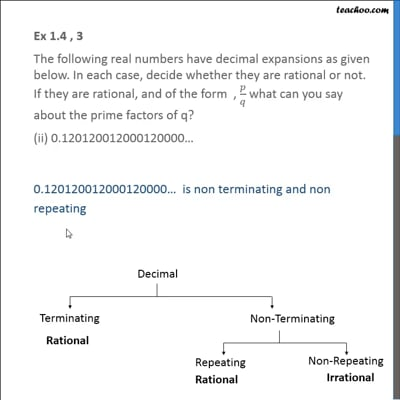This video is only available for Teachoo black users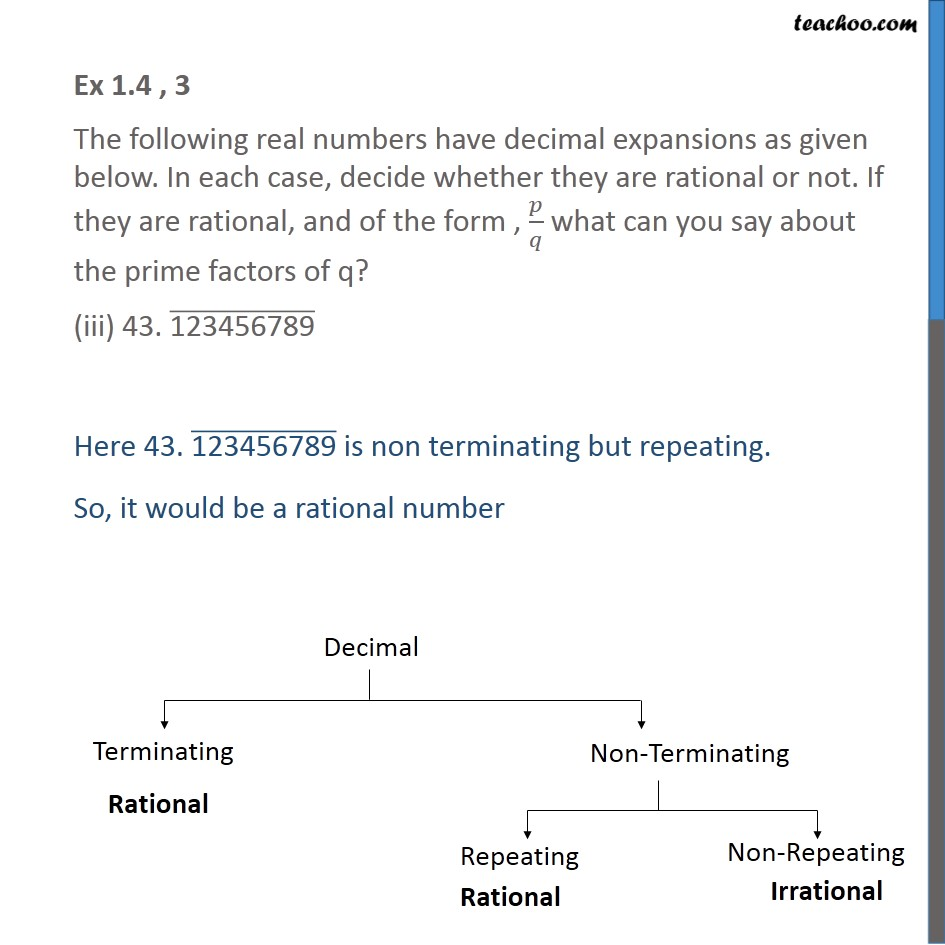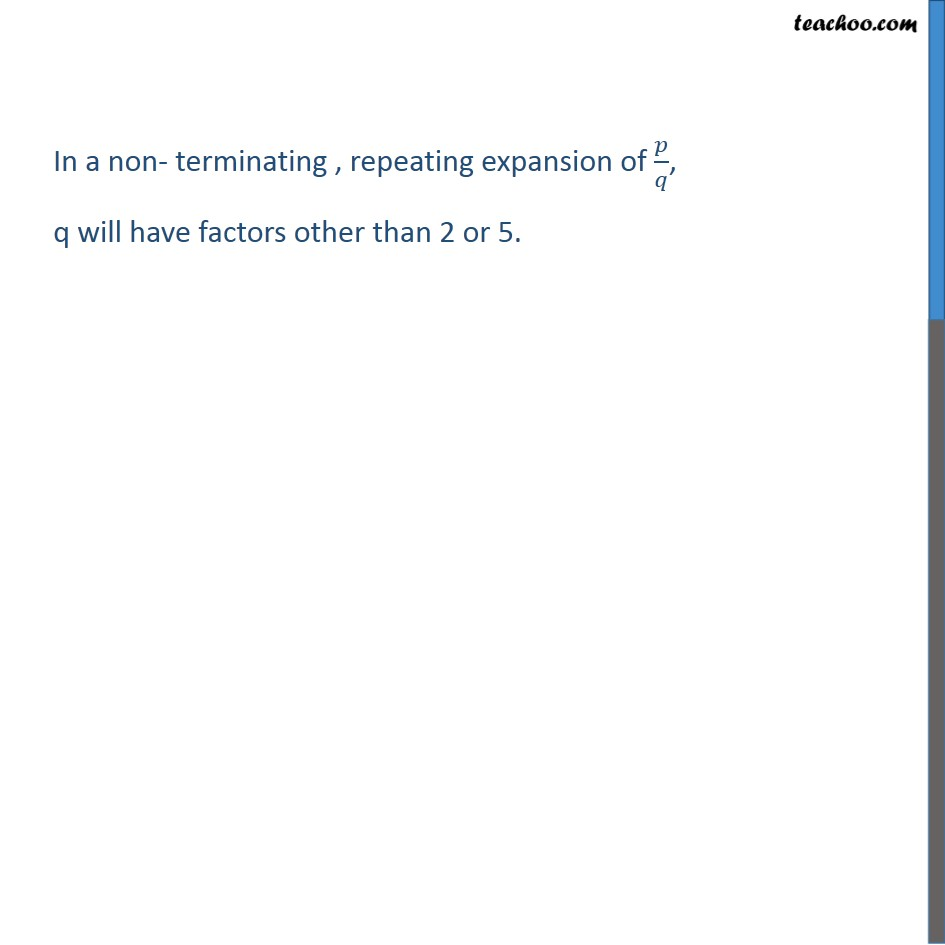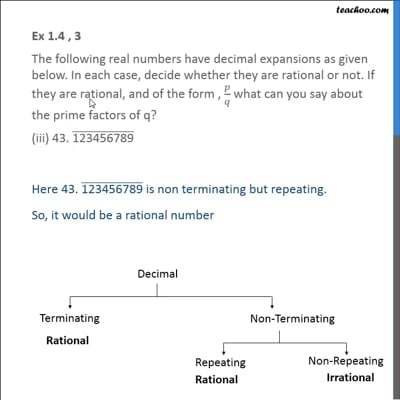This video is only available for Teachoo black users

Solve all your doubts with Teachoo Black (new monthly pack available now!)

### Transcript

Ex 1.4 , 3 (Method 1) The following real numbers have decimal expansions as given below. In each case, decide whether they are rational or not. If they are rational, and of the form , 𝑝/𝑞 what can you say about the prime factors of q? 43.123456789 43.123456789 is terminating So, it would be a rational number 43.123456789 = 43123456789/1000000000 = 43123456789/(10)9 = 43123456789/(2 ×5)9 = 43123456789/(29 ×59) Hence 43.123456789 is now in the form of 𝑝/𝑞 And the prime factors of q are in terms of 2 and 5 Ex 1.4 , 3 (Method 2) The following real numbers have decimal expansions as given below. In each case, decide whether they are rational or not. If they are rational, and of the form , 𝑝/𝑞 what can you say about the prime factors of q? 43.123456789 43.123456789 is terminating So, it would be a rational number In a terminating expansion of 𝑝/𝑞, q is of the form 2n 5m So, prime factors of q will be 2 or 5 or both only. Ex 1.4 , 3 The following real numbers have decimal expansions as given below. In each case, decide whether they are rational or not. If they are rational, and of the form , 𝑝/𝑞 what can you say about the prime factors of q? (ii) 0.120120012000120000… 0.120120012000120000… is non terminating and non repeating So, it is not a rational number Ex 1.4 , 3 The following real numbers have decimal expansions as given below. In each case, decide whether they are rational or not. If they are rational, and of the form , 𝑝/𝑞 what can you say about the prime factors of q? (iii) 43. ("123456789" ) ̅ Here 43. ("123456789" ) ̅ is non terminating but repeating. So, it would be a rational number In a non- terminating , repeating expansion of 𝑝/𝑞, q will have factors other than 2 or 5.